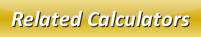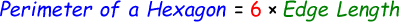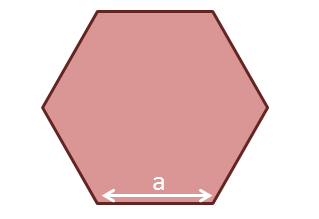# Perimeter of a Hexagon Calculator

The perimeter of a hexagon is the path which surrounds the hexagon shape from the outside surface. We can calculate perimeter of a hexagon with the help of this below formula:From the above formula, the edge length (a) is the distance from one edge to the other edge point of the hexagon as shown below:In the below online perimeter of a hexagon calculator, enter the edge length and then click calculate to find the hexagon perimeter.

 Edge Length: Perimeter of Hexagon:

Latest Calculator Release

Average Acceleration Calculator

Average acceleration is the object's change in speed for a specific given time period. ...

Free Fall Calculator

When an object falls into the ground due to planet's own gravitational force is known a...

Torque Calculator

Torque is nothing but a rotational force. In other words, the amount of force applied t...

Average Force Calculator

Average force can be explained as the amount of force exerted by the body moving at giv...

Angular Displacement Calculator

Angular displacement is the angle at which an object moves on a circular path. It is de...